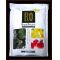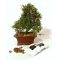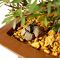##### The Japanese Bonsai specialist
Direct order Contact Help / Services Newsletter# Biogold original bonsai fertiliser 1 bag 900 gr

› Bonsai soil and fertiliser › Fertilizerref. : 5501

21,00

Available quantity : 39Order

###### Description

The best natural bonsai manure currently in vogue in Japan. This is for feeding trees when they're at their peak and in full bloom. The fertiliser is extremely versatile and can be scattered directly onto the earth, added to water in your watering can or mixed with earth for repotting.

1 sachet of 900 grams. Nitrogen, phosphorus, potassium content N .P .K 5.5-6.5-3.5

How to use:

1. Distribute across the whole surface of the earth, from the trunk to the side of the pot. Use approximately once per month from May to September. For a pot of 15 cm use 5 to 8 grains.

2. Dissolve in water at a rate of 2 to 3 grains per litre of water and water your trees and plants every 10 days.

3. Mix it into earth for repotting. Add 3 grains to 1 litre of earth.

This manure remains efficient for about 40 to 45 days. The odour will disappear after the first watering. It doesn't attract birds, slugs or worms. The triangular pellets do not roll on the soil.

#earth 5.5 #water 4.5 #fertiliser 4 #grains 3.6 #bonsai 3.6 #fertilizer 3 #repotting 2.9 #watering 2.8 #original 2.8 #biogold 2.7

Formule
(( ROUND((CHAR_LENGTH(b.article_nom)-CHAR_LENGTH(REPLACE(b.article_nom, 'earth', '')))/LENGTH('earth')) + ROUND((CHAR_LENGTH(b.article_description)-CHAR_LENGTH(REPLACE(b.article_description, 'earth', '')))/LENGTH('earth')) ) * 5.5) + (( ROUND((CHAR_LENGTH(b.article_nom)-CHAR_LENGTH(REPLACE(b.article_nom, 'water', '')))/LENGTH('water')) + ROUND((CHAR_LENGTH(b.article_description)-CHAR_LENGTH(REPLACE(b.article_description, 'water', '')))/LENGTH('water')) ) * 4.5) + (( ROUND((CHAR_LENGTH(b.article_nom)-CHAR_LENGTH(REPLACE(b.article_nom, 'fertiliser', '')))/LENGTH('fertiliser')) + ROUND((CHAR_LENGTH(b.article_description)-CHAR_LENGTH(REPLACE(b.article_description, 'fertiliser', '')))/LENGTH('fertiliser')) ) * 4) + (( ROUND((CHAR_LENGTH(b.article_nom)-CHAR_LENGTH(REPLACE(b.article_nom, 'grains', '')))/LENGTH('grains')) + ROUND((CHAR_LENGTH(b.article_description)-CHAR_LENGTH(REPLACE(b.article_description, 'grains', '')))/LENGTH('grains')) ) * 3.6) + (( ROUND((CHAR_LENGTH(b.article_nom)-CHAR_LENGTH(REPLACE(b.article_nom, 'bonsai', '')))/LENGTH('bonsai')) + ROUND((CHAR_LENGTH(b.article_description)-CHAR_LENGTH(REPLACE(b.article_description, 'bonsai', '')))/LENGTH('bonsai')) ) * 3.6) + (( ROUND((CHAR_LENGTH(b.article_nom)-CHAR_LENGTH(REPLACE(b.article_nom, 'repotting', '')))/LENGTH('repotting')) + ROUND((CHAR_LENGTH(b.article_description)-CHAR_LENGTH(REPLACE(b.article_description, 'repotting', '')))/LENGTH('repotting')) ) * 2.9) + (( ROUND((CHAR_LENGTH(b.article_nom)-CHAR_LENGTH(REPLACE(b.article_nom, 'watering', '')))/LENGTH('watering')) + ROUND((CHAR_LENGTH(b.article_description)-CHAR_LENGTH(REPLACE(b.article_description, 'watering', '')))/LENGTH('watering')) ) * 2.8) + (( ROUND((CHAR_LENGTH(b.article_nom)-CHAR_LENGTH(REPLACE(b.article_nom, 'original', '')))/LENGTH('original')) + ROUND((CHAR_LENGTH(b.article_description)-CHAR_LENGTH(REPLACE(b.article_description, 'original', '')))/LENGTH('original')) ) * 2.8) + (( ROUND((CHAR_LENGTH(b.article_nom)-CHAR_LENGTH(REPLACE(b.article_nom, 'biogold', '')))/LENGTH('biogold')) + ROUND((CHAR_LENGTH(b.article_description)-CHAR_LENGTH(REPLACE(b.article_description, 'biogold', '')))/LENGTH('biogold')) ) * 2.7) + (( ROUND((CHAR_LENGTH(b.article_nom)-CHAR_LENGTH(REPLACE(b.article_nom, 'manure', '')))/LENGTH('manure')) + ROUND((CHAR_LENGTH(b.article_description)-CHAR_LENGTH(REPLACE(b.article_description, 'manure', '')))/LENGTH('manure')) ) * 2.6)

## Secure payment## Delivery

Our logistic partners :